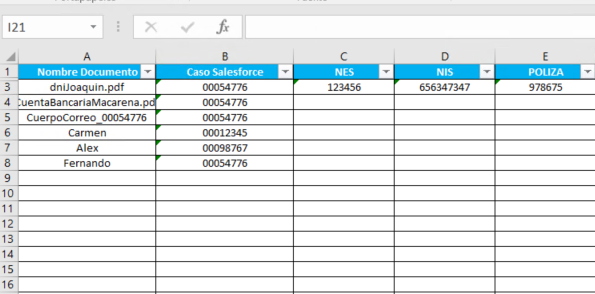# A way to copy one cell value to several cells in an Excel Sheet

Hello!

I have a process that I am finding quite complicated. Let me put you in situation:

I receive daily documents via email which are automatically assigned a salesforce identifier (column B). These emails are usually associated with a series of unique IDs: NES, NIS, POLIZA (columns C, D, E respectively).

As you can see in the image, the first row already has these IDs. I would like to know if there is a way to copy those IDs to all the rows that have the same salesforce ID (column B). In the example in the picture, it would be to copy the values NES 123456, NIS 656347347 and POLICY 978675 to rows 4, 5 and 8.Any idea? Thank you very much!

Yes, there is a way to copy the values from the unique IDs (columns C, D, and E) to all the rows that have the same Salesforce ID (column B) in Excel.

One way to accomplish this is by using the VLOOKUP function. In the cell where you want the unique ID to be copied, you can use the formula =VLOOKUP(B4,A1:E3,C4,FALSE) for column C, =VLOOKUP(B4,A1:E3,D4,FALSE) for column D and =VLOOKUP(B4,A1:E3,E4,FALSE) for column E. Replace the range A1:E3 with the range where your data is located and the number in the last argument with the number of the column where the value you want to copy is located.

HI,

How about the following using Dictionary with InvokeCode?

``````Dim dict As Dictionary(Of String, Object())
dict = dt.AsEnumerable.GroupBy(Function(r) r("Caso Salesforce").ToString).ToDictionary(Function(g) g.Key,Function(g) g.First.ItemArray)
dt.AsEnumerable.ToList.ForEach(
Sub(r)
r.ItemArray = {r(0),r(1)}.Concat(dict(r(1).ToString).Skip(2)).ToArray
End Sub
)
``````

Sample20230120-6L.zip (9.9 KB)

Regards,

Hello Alejandro,

Would you be open to using a vb.net solution for the same :
here is the psudo codeDim lastRow as String
lastRow = ws.UsedRange.rows.count.Tostring()
ws.Range(“C4:C”+lastRow).value = ws.range(“C3”).value

This should do the trick
Hope it helps…

Regards
Ankit

This topic was automatically closed 3 days after the last reply. New replies are no longer allowed.